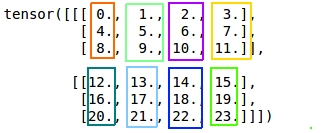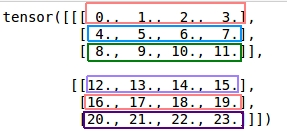# How to implement dim parameter of torch.max()?

Hi all, I am trying to implement a variation of `torch.max()` and confused about the implementation of `dim` parameter. Here is an example,

``````inp = torch.arange(0., 24).view(2, 3, 4)
torch.max(inp, dim=1)

torch.return_types.max(
values=tensor([[ 8.,  9., 10., 11.],
[20., 21., 22., 23.]]),
indices=tensor([[2, 2, 2, 2],
[2, 2, 2, 2]]))
``````

So, the max value within each colored rectangle is returned as in the figure.Likewise, `torch.max(inp, dim=2)` returns the max value of each colored rectangle in the below figure,

``````torch.return_types.max(
values=tensor([[ 3.,  7., 11.],
[15., 19., 23.]]),
indices=tensor([[3, 3, 3],
[3, 3, 3]]))
``````My question is how can I reshape the input as done with the `torch.max()` in order to use `dim` parameter in my custom max implementation ? I’ve experimented `view` with different values but it didn’t work so far.

What is your custom `max` operation doing differently and what is not working at the moment?

The custom `max` should return the indices of all maximum values instead of the first one being encountered as in `torch.max`. I want to add `dim` as a parameter to my custom `max`, like in `torch.max`. But I don’t know how to implement the dimension reduction effect controlled by `dim`.

Documentation of `torch.max` states as below but the input is not in the shape of `(Ax1xB)`, so using `torch.squeeze` does not yield to reduced dimensions.

Otherwise, dim is squeezed (see `torch.squeeze()`), resulting in the output tensors having 1 fewer dimension than input

If you want to return more than a single `max` value, you could try to use e.g. `topk` instead of `max` or sort the tensor and grab the values manually.

The documentation of `topk` says it returns the k largest elements but I only need the max values and don’t know how many of them will be there.

I tried using `max` but it results in only the first max value having the gradient, it’s not shared among the max values. Here is an example,

``````inp = torch.tensor([[ 6.,  2,  3,  6],
[ 9, 16, 16, 16]], requires_grad=True)
outp, inds = torch.max(inp, dim=-1)
outp.sum().backward()
print('outp:\n', outp)

outp:
tensor([[1., 0., 0., 0.],
[0., 1., 0., 0.]])
``````

I expect the gradient to be like below,

``````inp.grad:
tensor([[0.5, 0., 0., 0.5],
[0., 0.333, 0.333, 0.333]])
``````

So, I think I have to implement a custom `max` but I don’t know how to reshape the input (or squeeze it) controlled by the `dim` parameter in `torch.max`.

Thanks for the follow-up as I misunderstood your use case.
Based on your description it seems that the returned values would have a variable shape. E.g. one window could have the same max values, while another one a single one.
If that’s the case I think you would have to return a `list` of these values e.g. by getting the max value via `torch.max` and checking if duplicates can be found in the current window.

I’m not sure how you would like to further process the result `list`, as you wouldn’t be able to create a single return tensor (with nested tensors this might be possible).

Thank you for bearing with me, this post will be a bit long. The returned values by `max` don’t need to be variable in shape since they keep the max value and there is only one (maybe repeating) max within the window, but the returned indices will be variable in shape. An example is at the `MultiMax` part.

I’ve implemented the custom `max` (named `MultiMax`) as a custom autograd function and the max pooling (named `MultiMaxPool2d`) layer using it. As I asked in this thread, I don’t know how to implement the `dim` parameter of `torch.max` for my `MultiMax`. So, `MultiMax` takes a 1d tensor and is called within the `for` loop of `MultiMaxPool2d` layer. I’ve just learned this approach might be problematic since in-place operations should be avoided in PyTorch.

My question is, what should be the proper approach instead of using the in-place operations for creating the pooled output ? I obviously need to select max values from the input and assign them to their new positions in the pooled output. I’ve added the source code and examples.

Here is my custom `max` operation `MultiMax`,

``````import torch

@staticmethod
def forward(ctx, input):
mmax = torch.max(input)
inds = torch.nonzero(mmax == input).squeeze() # Get max inds
ctx.save_for_backward(input, mmax, inds)
ctx.mark_non_differentiable(inds)
return mmax, inds

@staticmethod
input, mmax, inds = ctx.saved_tensors
inds_shape = 1 if inds.dim() == 0 else inds.shape
``````

An example using `MultiMax`,

``````inp = torch.tensor([6., 2, 5, 6], requires_grad=True)
mmax = MultiMax.apply
pooled, inds = mmax(inp)
pooled.sum().backward()
print('pooled:\n', pooled)
print('inds:\n', inds)

pooled:
inds:
tensor([0, 3])
tensor([0.5000, 0.0000, 0.0000, 0.5000])
``````

Here is the implementation of custom max pooling `MultiMaxPool2d` using `MultiMax` (adapted from here),

``````import torch
import torch.nn as nn
import torch.nn.functional as F
from MultiMax import MultiMax

class MultiMaxPool2d(nn.Module):

def __init__(self, kernel_size, stride, padding=0, same=False):
super(MultiMaxPool2d, self).__init__()
self.k = kernel_size if type(kernel_size) is tuple else _pair(kernel_size)
self.stride = stride if type(stride) is tuple else _pair(stride)
self.same = same

def _init_pool_inds(self, s):
pool = torch.zeros(s, s, s, s)
inds = []
for x in range(0, s):
inds.append([])
for y in range(0, s):
inds[x].append([])
for z in range(0, s):
inds[x][y].append([])
for t in range(0, s):
inds[x][y][z].append(0)
return pool, inds

if self.same:
ih, iw = x.size()[2:]
if ih % self.stride == 0:
ph = max(self.k - self.stride, 0)
else:
ph = max(self.k - (ih % self.stride), 0)
if iw % self.stride == 0:
pw = max(self.k - self.stride, 0)
else:
pw = max(self.k - (iw % self.stride), 0)
pl = pw // 2
pr = pw - pl
pt = ph // 2
pb = ph - pt
padding = (pl, pr, pt, pb)
else:

def forward(self, x):
x = x.unfold(2, self.k, self.stride).unfold(3, self.k, self.stride)
x = x.contiguous().view(x.size()[:4] + (-1,))
mmax = MultiMax.apply
s = x.shape[:4]
pool, inds = self._init_pool_inds(s)
for i in range(0, s):
for j in range(0, s):
for k in range(0, s):
for l in range(0, s):
_max, _is = mmax(x[i][j][k][l])
pool[i][j][k][l] = _max
inds[i][j][k][l] = _is
return pool, inds
``````

An example using `MultiMaxPool2d`,

``````inp = torch.tensor([[[[ 6.,  2,  3,  9],
[ 5,  6,  7,  8],
[ 9, 10, 11, 16],

mp = MultiMaxPool2d(kernel_size=2, stride=2)
pooled, inds = mp(inp)
pooled.sum().backward()
print('pooled:\n', pooled)
print('inds:\n', inds)

pooled:
tensor([[[[ 6.,  9.],
@ptrblck I still don’t know how to implement the dimension reduction for my custom `max` operation as controlled by the `dim` parameter of `torch.max` but this reply made it clear that if the code runs without errors, then autograd handles the in-place operations.